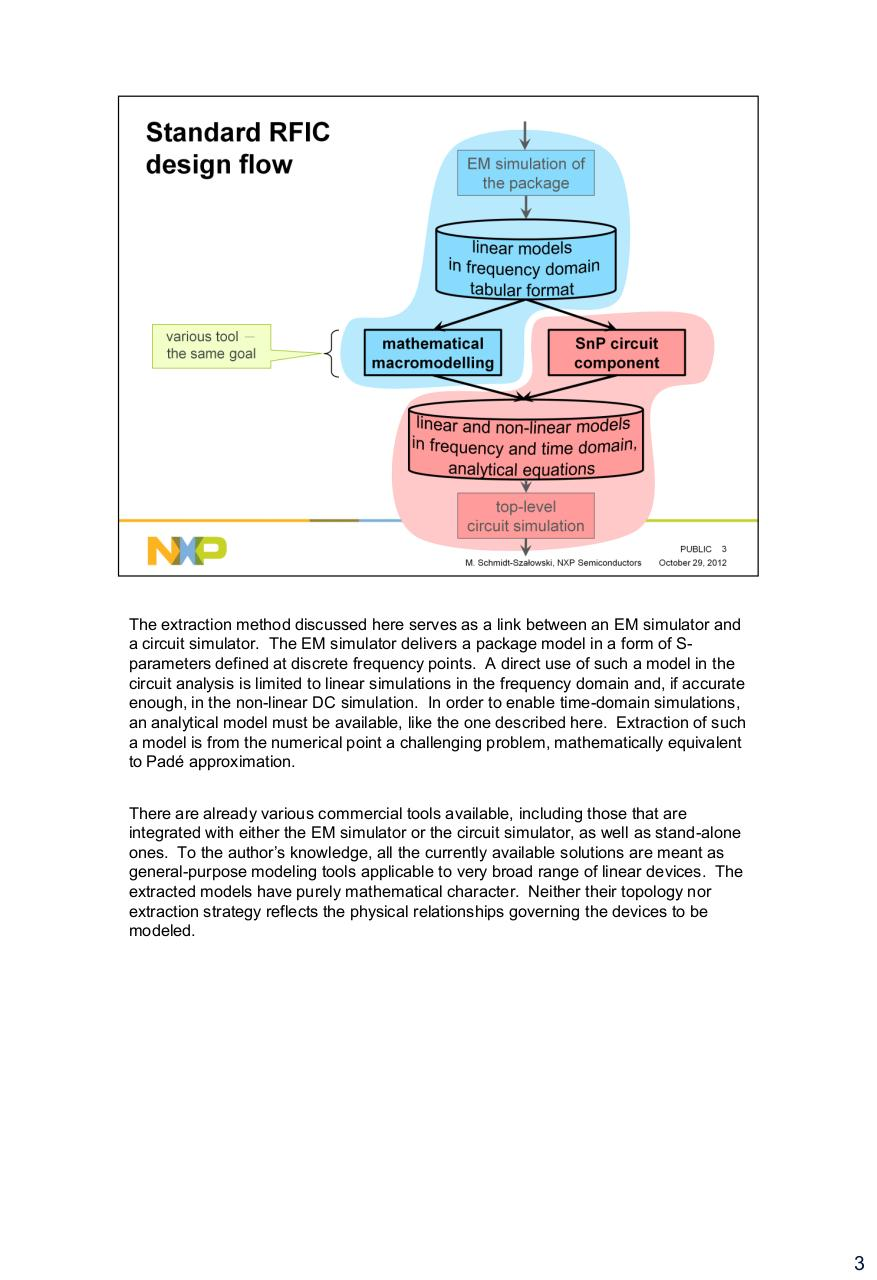# wideband spice compatible modeling of packages schmidt szalowski nxp slides with notes.pdfPage 1 2 34524

#### Text preview

The extraction method discussed here serves as a link between an EM simulator and
a circuit simulator. The EM simulator delivers a package model in a form of Sparameters defined at discrete frequency points. A direct use of such a model in the
circuit analysis is limited to linear simulations in the frequency domain and, if accurate
enough, in the non-linear DC simulation. In order to enable time-domain simulations,
an analytical model must be available, like the one described here. Extraction of such
a model is from the numerical point a challenging problem, mathematically equivalent